# GMAT Math : Circles

## Example Questions

← Previous 1 3 4 5 6 7 8 9

### Example Question #1 : Dsq: Calculating The Area Of A Circle

You are given a circle and a square. Which one has the larger area?

Statement 1: The radius of the circle is two-thirds the sidelength of the square.

Statement 2: The circumference of the circle is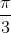times the perimeter of the square.

Statement 1 ALONE is sufficient to answer the question, but Statement 2 ALONE is NOT sufficient to answer the question.

EITHER statement ALONE is sufficient to answer the question.

BOTH statements TOGETHER are insufficient to answer the question.

BOTH statements TOGETHER are sufficient to answer the question, but NEITHER statement ALONE is sufficient to answer the question.

Statement 2 ALONE is sufficient to answer the question, but Statement 1 ALONE is NOT sufficient to answer the question

EITHER statement ALONE is sufficient to answer the question.

Explanation:

Letbe the sidelength of the square. Then its area is.

From Statement 1, it follows that the radius of the circle is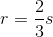From Statement 2,  it follows that , since the perimeter of the square is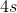, the circumference of the circle is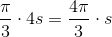, and the radius is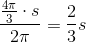- the same fact given in Statement 1.

Either way, it follows that the area of the circle in terms ofis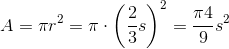,

so all we have to do is compare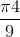to 1 in order to determine whether the square or the circle is larger in area.

### Example Question #1 : Circles

You are given a circle and an equilateral triangle. Which one has the greater area?

Statement 1: The sidelength of the triangle is three times the radius of the circle.

Statement 2: The perimeter of the triangle is 99 inches.

Statement 1 ALONE is sufficient to answer the question, but Statement 2 ALONE is NOT sufficient to answer the question.

EITHER statement ALONE is sufficient to answer the question.

Statement 2 ALONE is sufficient to answer the question, but Statement 1 ALONE is NOT sufficient to answer the question.

BOTH statements TOGETHER are sufficient to answer the question, but NEITHER statement ALONE is sufficient to answer the question.

BOTH statements TOGETHER are insufficient to answer the question.

Statement 1 ALONE is sufficient to answer the question, but Statement 2 ALONE is NOT sufficient to answer the question.

Explanation:

From Statement 2, we can calculate the area of the triangle, but we are given no clues about the area of the circle, actual or relative.

From Statement 1, we know that if we call the radius of the circle, we know the sidelength of the triangle is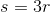.

The area of the circle is.

The area of the triangle is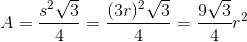.

All we have to do is compareto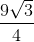to determine whether the circle or the triangle has the greater area.

### Example Question #1 : Radius

Data Sufficiency Question

Calculate the area of a circle.

1. The radius of the circle is 4.

2. The circumference of the circle is 24.

Each statement alone is sufficient

Both statements taken together are sufficient to answer the question, but neither statement alone is sufficient

Statement 2 alone is sufficient, but statement 1 alone is not sufficient to answer the question

Statement 1 alone is sufficient, but statement 2 alone is not sufficient to answer the question

Statements 1 and 2 together are not sufficient, and additional data is needed to answer the question

Each statement alone is sufficient

Explanation:

The area of a circle can be calcuated using the equation: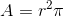and the circumference calculated using: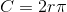The radius is the only information required for calculating the area of a circle and that can be obtained from the circumference, therefore, either statement is sufficient.

### Example Question #1 : Radius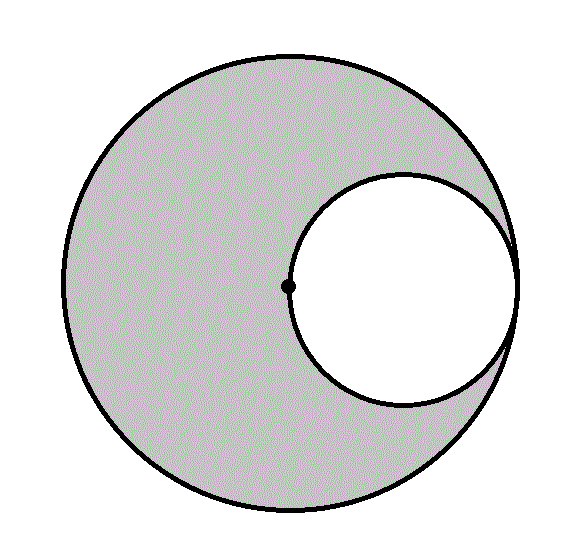What is the area of the gray region in the above figure?

Statement 1: The diameter of the larger circle is one mile.

Statement 2: The radius of the smaller circle is 1,320 feet.

BOTH statements TOGETHER are sufficient to answer the question, but NEITHER statement ALONE is sufficient to answer the question.

Statement 2 ALONE is sufficient to answer the question, but Statement 1 ALONE is NOT sufficient to answer the question.

Statement 1 ALONE is sufficient to answer the question, but Statement 2 ALONE is NOT sufficient to answer the question.

BOTH statements TOGETHER are insufficient to answer the question.

EITHER statement ALONE is sufficient to answer the question.

EITHER statement ALONE is sufficient to answer the question.

Explanation:

The radius of the larger circle is equal to the diameter of the smaller, and, subsequently, twice the radius of the smaller. If one radius is known, then the other can be calculated, and the areas of the two circles can be as well; the difference between their areas is the area of the gray region. Statement 1 tells us the diameter of the large circle, from which its radius can be determined by dividing by 2; Statement 2 tells us the radius of the radius of the smaller circle. From either, the radius of the other circle can be calculated.

### Example Question #5 : Radius

Two circles are constructed; one is inscribed inside a given regular hexagon, and the other is circumscribed about the same hexagon.

What is the area of the inscribed circle?

Statement 1: The area of the circumscribed circle is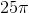Statement 2: The perimeter of the hexagon is 30.

EITHER statement ALONE is sufficient to answer the question.

Statement 2 ALONE is sufficient to answer the question, but Statement 1 ALONE is NOT sufficient to answer the question.

BOTH statements TOGETHER are sufficient to answer the question, but NEITHER statement ALONE is sufficient to answer the question.

Statement 1 ALONE is sufficient to answer the question, but Statement 2 ALONE is NOT sufficient to answer the question.

BOTH statements TOGETHER are insufficient to answer the question.

EITHER statement ALONE is sufficient to answer the question.

Explanation:

Examine the diagram below, which shows the hexagon, segments from its center to two vertices and the midpoint of a side, and the two circles.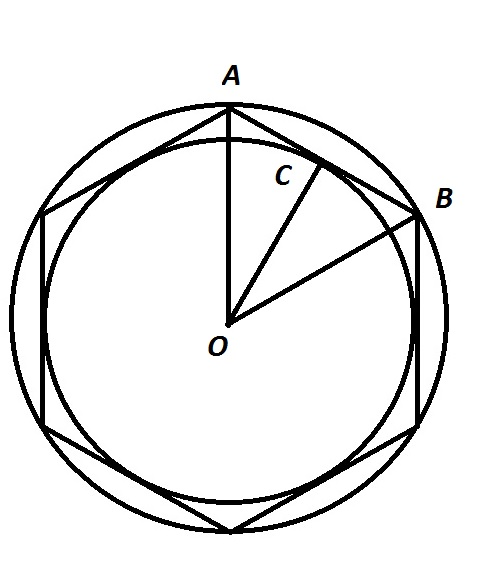From Statement 1 alone, the radius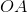of the circumscribed circle can be calculated using the area formula. From Statement 2 alone, the perimeter can be divided by 6 to obtain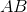; since two consecutive radii and one side of a hexagon can be proved to form an equilateral triangle,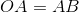. As a consequence, from either statement alone,can be calculated.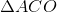can be proved a 30-60-90 triangle, so the 30-60-90 Theorem can be used to calculate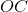, the radius of the inscribed circle. From this, the area of the inscribed circle can be calculated.

### Example Question #6 : Radius

The equation of a given circle is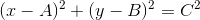.

What is the radius of the circle?

Statement 1: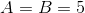Statement 2: The circle passes through the origin.

Statement 1 ALONE is sufficient to answer the question, but Statement 2 ALONE is NOT sufficient to answer the question.

EITHER statement ALONE is sufficient to answer the question.

BOTH statements TOGETHER are sufficient to answer the question, but NEITHER statement ALONE is sufficient to answer the question.

Statement 2 ALONE is sufficient to answer the question, but Statement 1 ALONE is NOT sufficient to answer the question.

BOTH statements TOGETHER are insufficient to answer the question.

BOTH statements TOGETHER are sufficient to answer the question, but NEITHER statement ALONE is sufficient to answer the question.

Explanation:

The standard form of the equation of a circle is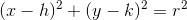,

where the radius isand the center is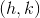.

The equation given is this same form, withreplacing,replacing, andreplacing, so to find the radius, we need to findStatement 1 alone tells us that the center is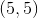but it tells us nothing about the radius. Statement 2 alone tells us only that the circle passes through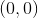The two together, however, reveal enough information to give the radius. The radius is the distance from the center to a point on the circle, so we can use the distance formula to find the distance betweenand. This is the radius.

### Example Question #7 : Radius

Find the radius of circle B

I) Circle B has a circumference of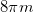.

II) Circle B has an area of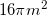.

Statement 1 is sufficient to solve the question, but statement 2 is not sufficient to solve the question.

Statement 2 is sufficient to solve the question, but statement 1 is not sufficient to solve the question.

Neither statement is sufficient to solve the question. More information is needed.

Both statements taken together are sufficient to solve the question.

Each statement alone is enough to solve the question.

Each statement alone is enough to solve the question.

Explanation:

We are given the area and circumference of a circle and asked to find the radius.

Given the following equations: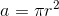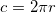We can use either equation to work backwards and find our radius, therefore; Each statement alone is enough to solve the question.

### Example Question #1 : Dsq: Calculating The Length Of A Radius

Calculate the length of the radius of a circle.

Statement 1):  The circumference of the circle is.

Statement 2):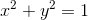Statement 1) ALONE is sufficient, but Statement 2) ALONE is not sufficient to answer the question.

Statement 2) ALONE is sufficient, but Statement 1) ALONE is not sufficient to answer the question.

EACH statement ALONE is sufficient.

BOTH statements TOGETHER are NOT sufficient, and additional data is needed to answer the question.

BOTH statements taken TOGETHER are sufficient to answer the question, but neither statement ALONE is sufficient.

EACH statement ALONE is sufficient.

Explanation:

Statement 1) gives the circumference of a circle.  The formula for finding the circumference of a circle is.  The radius can be solved by using this formula.

Statement 2) gives the standard form of a circle, whereis the center of the circle:The radius is also given in the equation.

Therefore, either statement alone is enough to solve for the radius of a circle.

### Example Question #1 : Dsq: Calculating The Length Of A Radius

Let circlerepresent the base of a lamp. Find its radius.

I) The ratio of circle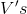diameter to circumference is approximately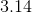.

II) The base of the lamp will cover an area of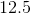square inches.

Both statements are needed to answer the question.

Statement I is sufficient to answer the question, but statement II is not sufficient to answer the question.

Either statement is sufficient to answer the question.

Statement II is sufficient to answer the question, but statement I is not sufficient to answer the question.

Statement II is sufficient to answer the question, but statement I is not sufficient to answer the question.

Explanation:

To find the radius of a cricle, we need its circumference, diameter, or area.

I) Seems to be helpful, but it is really just giving us pi, so it is not sufficient.

II) Gives us the area of the circle, which we can use to work backward to find the radius.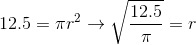So II is sufficient to answer the question, but I is not.

### Example Question #10 : Radius

Between Circle 1 and Circle 2, which has the greater radius?

Statement 1:  A right triangle, both of whose legs measures 10, can be inscribed inside Circle 1; a square of perimeter 48 can be inscribed inside Circle 2.

Statement 2: A square of area 100 can be inscribed inside Circle 1; a 30-60-90 triangle, one of whose legs measures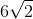, can be inscribed in side Circle 2.

BOTH statements TOGETHER are sufficient to answer the question, but NEITHER statement ALONE is sufficient to answer the question.

EITHER statement ALONE is sufficient to answer the question.

Statement 2 ALONE is sufficient to answer the question, but Statement 1 ALONE is NOT sufficient to answer the question.

BOTH statements TOGETHER are insufficient to answer the question.

Statement 1 ALONE is sufficient to answer the question, but Statement 2 ALONE is NOT sufficient to answer the question.

Statement 1 ALONE is sufficient to answer the question, but Statement 2 ALONE is NOT sufficient to answer the question.

Explanation:

The length of a diagonal of a square inscribed inside a circle is equal to the diameter of the circle, and half this is the radius. If a right triangle is inscribed inside a circle, The hypotenuse of a right triangle inscribed inside a circle is also a diameter, and half the length is the radius. Since each statement alone mentions an inscribed square in one circle to an inscribed right triangle in the other, we need only compare the length of a diagonal of the former to that of the hypotenuse of the latter.

Assume Statement 1 alone. The square inscribed inside Circle 2 has perimeter 48; its sidelength is one fourth this, or 12, and the length of a diagonal istimes this, or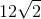. The hypotenuse of the right triangle inscribed in Circle 1, with both legs equal to 10 - which is a 45-45-90 triangle, being isosceles - is, by the 45-45-90 Theorem,times the length of a leg, or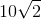. The diagonal of the square inscribed inside Circle 2 is longer than the hypotenuse of the triangle inscribed inside Circle 1, so Circle 2 has the greater radius.

Assume Statement 2 alone. A square of area 100 - and, subsequently, of sidelength the square root of this, or 10 - can be inscribed inside Circle 1; its diagonal will have lengththis, or.

The 30-60-90 triangle inscribed inside Circle 2 has a leg of length. However, Statement 2 does not make it clear whether the leg is the shorter leg or the longer leg. If it is the shorter leg, then by the 30-60-90 Theorem, the hypotenuse is twice, or; if it is the longer leg, then by the 30-60-90 Theorem, the hypotenuse is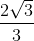times, or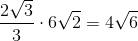.

In the first scenario, since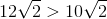, Circle 2 has the longer radius. In the second, since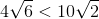(this can be seen by noting that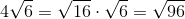and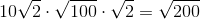), Circle 1 has the greater radius.

← Previous 1 3 4 5 6 7 8 9

### All GMAT Math Resources